Try our new search engine!

# Kindergarten Mathematics Worksheets

Mathematics worksheets designed for young learners in Kindergarten. These worksheets are for extra practice in the basic concepts of mathematics like adding and subtracting.#### Dot to Dot Numbers 1 – 10

A collection of tracing dot to dot worksheets on the numbers 1 - 10. Perfect for kindergarteners. Can colour it ...#### Kindergarten Mathematics: Count and Circle

A collection of 8 kindergarten mathematics worksheets focusing on the exercise, counting and circling. Young students will count the number ...A collection of addition worksheets for Kindergarten level! 5 worksheets in total. For more useful mathematics worksheets for young learners, ...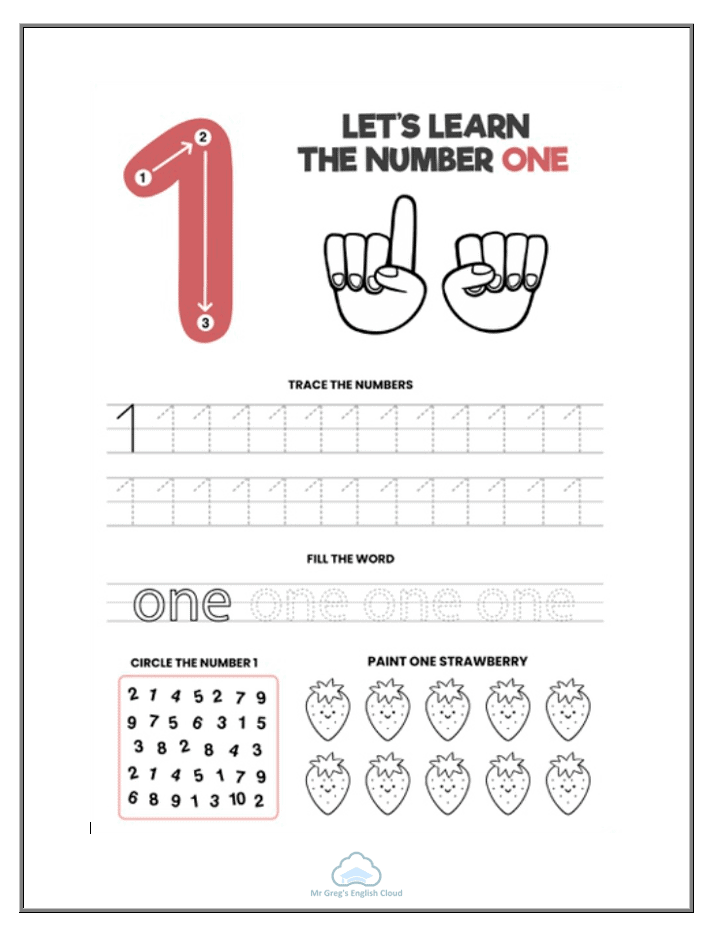#### Numbers 1 – 10 Booklet

The numbers 1 - 10 booklet is designed for Kindergarten students aged between 3 - 4 years old. Every page ...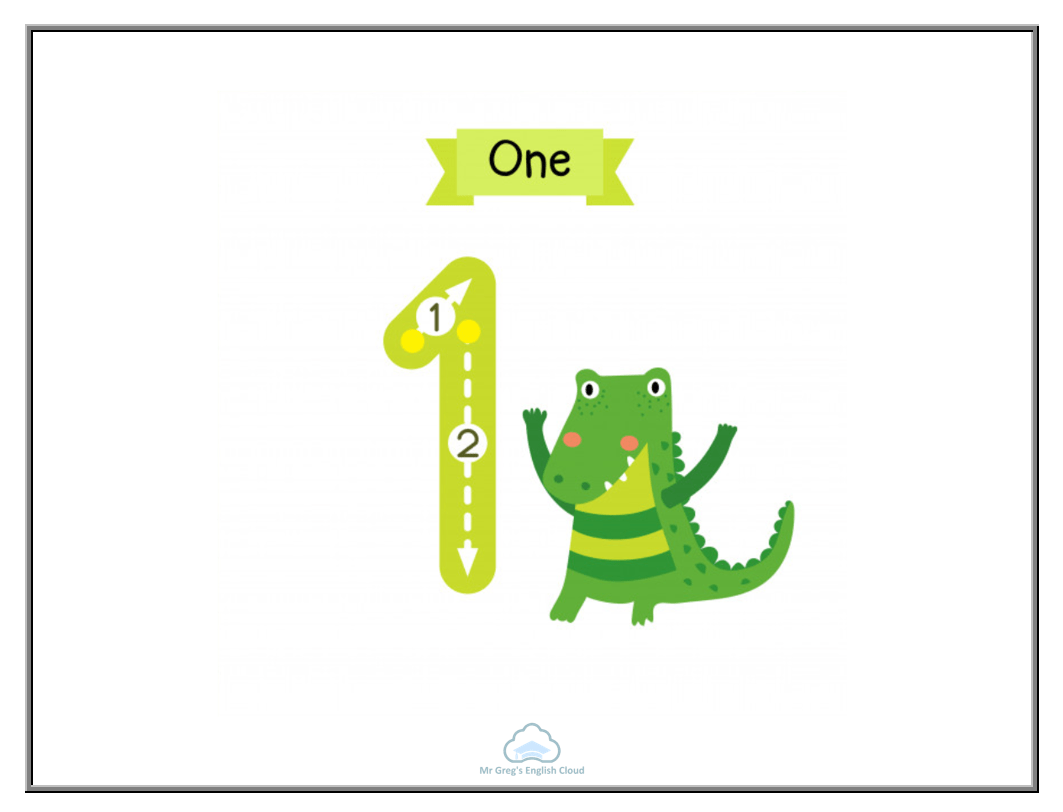#### Numbers 1 – 10 Writing Flashcards

Flashcards showing students how to write the numbers 1 - 10. Great to use as a wall filler or you ...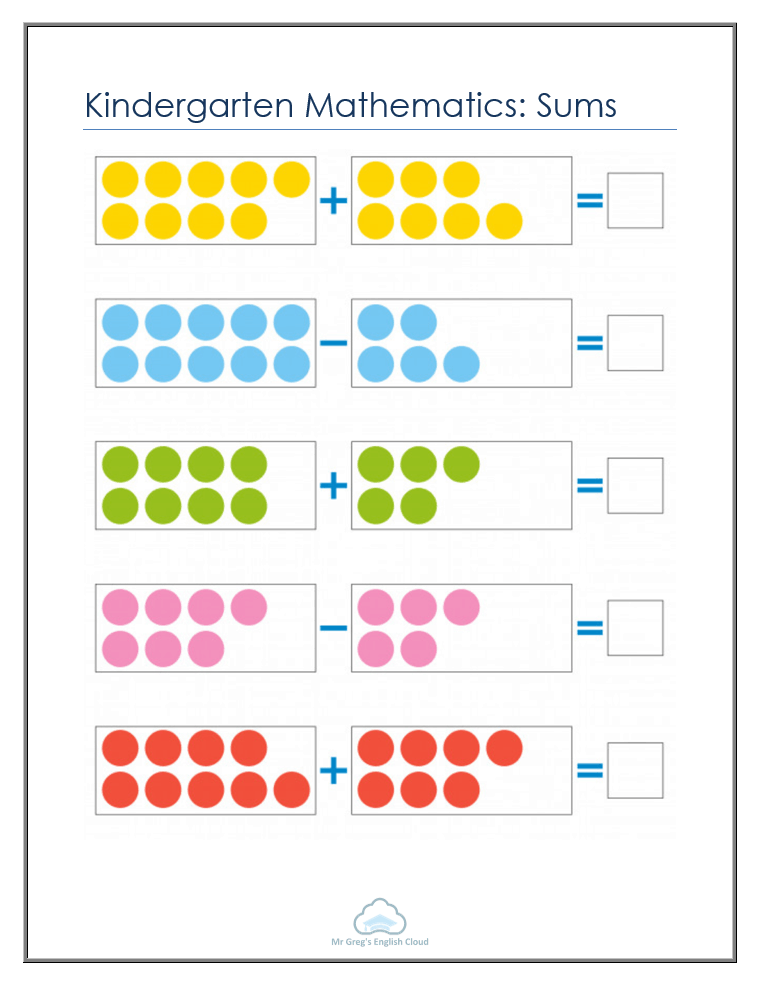#### Kindergarten Mathematics: Sums

Kindergarten mathematics worksheets on the topic of sums. Students will count the amount of circles in the rectangles, complete the ...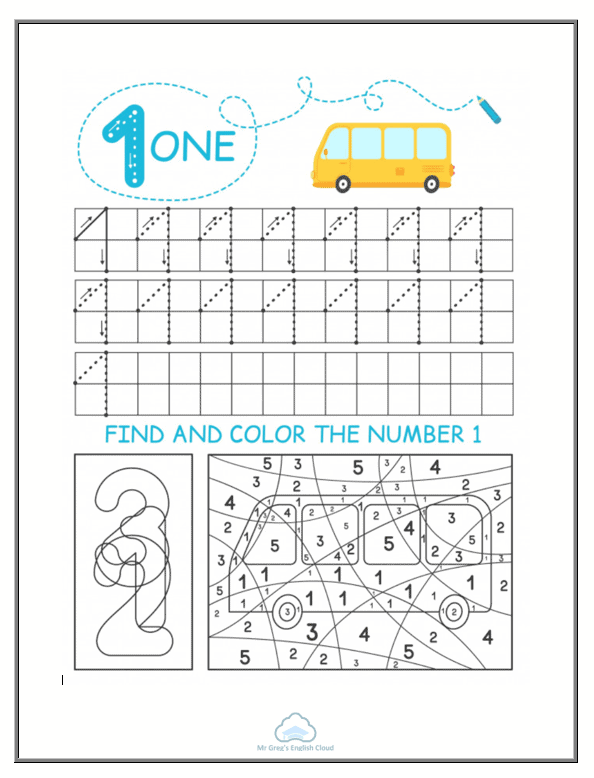#### Kindergarten Mathematics: 1 – 10

Kindergarten mathematics worksheets on the topic of 1 - 10. Students will trace the number and complete colouring activities to ...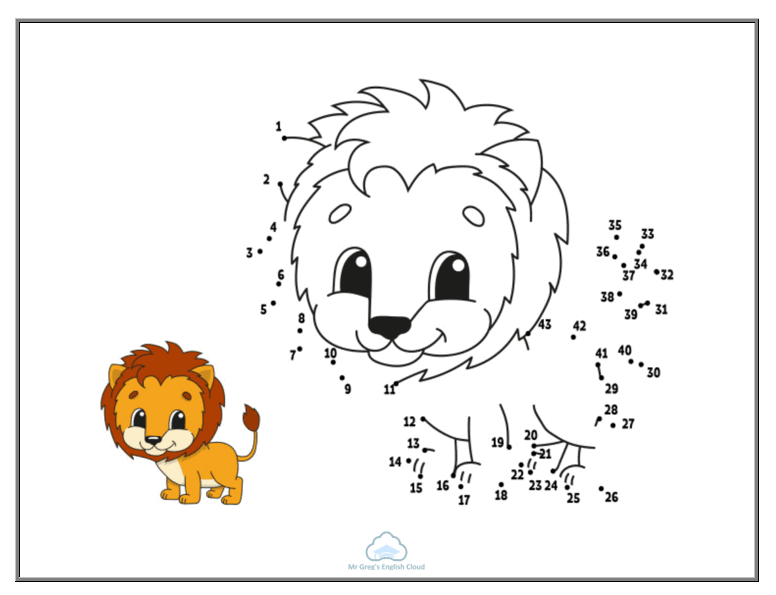#### Kindergarten Mathematics: Connect the Dots

Kindergarten mathematics worksheets on the topic of connect the dots. Students will join the dots in numerical order. 7 worksheets ...#### Kindergarten Mathematics: Missing Numbers

Kindergarten mathematics worksheets on the topic of missing numbers. Students can either cut out the numbers and stick them in ...#### Kindergarten Mathematics: How Many?

Kindergarten mathematics worksheets on the topic of how many. Students will count the coloured shapes in each worksheet and write ...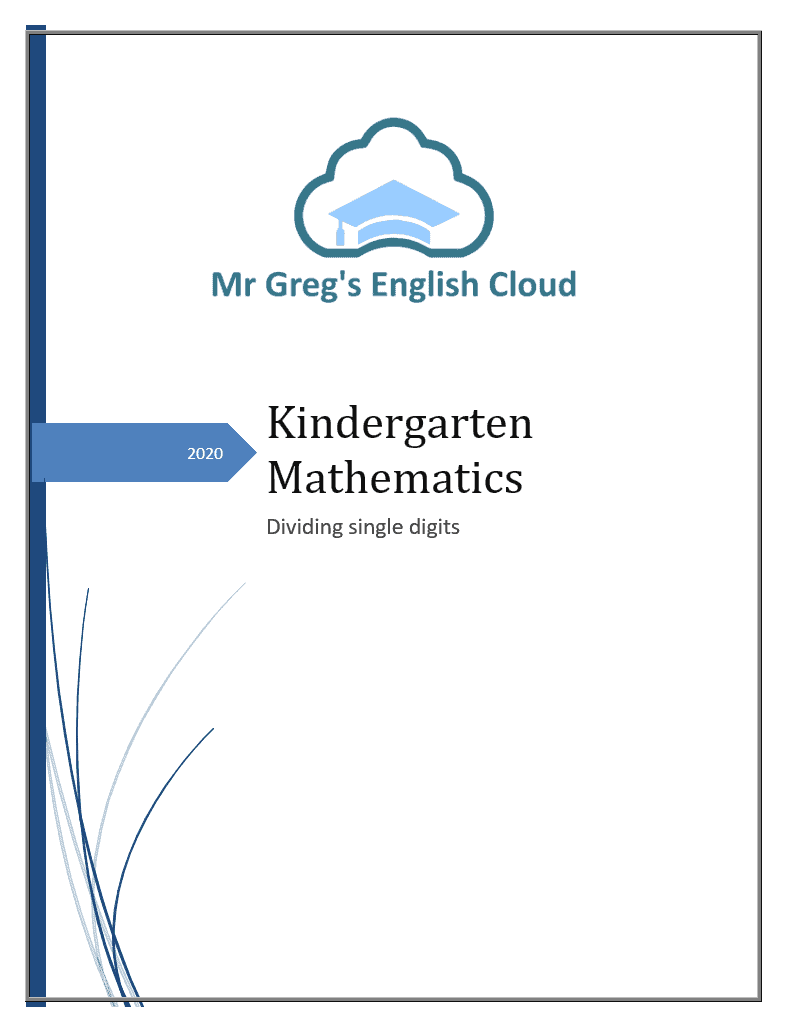#### Kindergarten Mathematics: Dividing

Kindergarten Mathematics workbook focusing on dividing single digits. Covers all divisions from numbers 1 to 9. Over 20 pages of ...#### Kindergarten Mathematics: Subtracting

Kindergarten Mathematics workbook focusing on subtracting single digits. Covers all additions from numbers 1 to 9. Over 20 pages of ...#### Kindergarten Mathematics: Multiplying

Kindergarten Mathematics workbook focusing on multiplying single digits. Covers all multiplications from numbers 1 to 9. Over 30 pages of ...#### Kindergarten Mathematics: Adding Single Digits

Kindergarten Mathematics workbook focusing on adding single digits. Covers all additions from numbers 1 to 9. Over 30 pages of ...

For more worksheets: http://www.mrgregenglish.com/mr-gregs-worksheets/